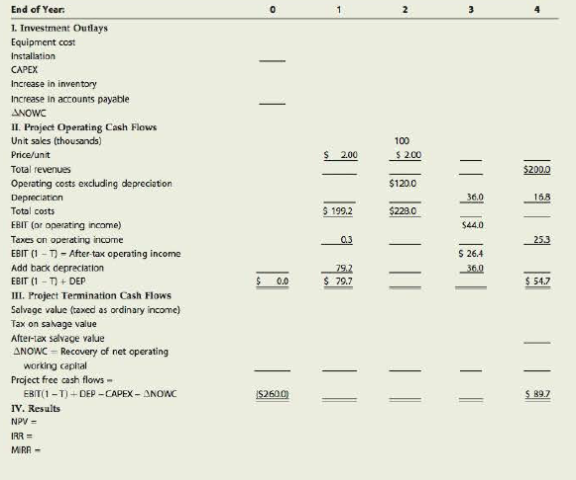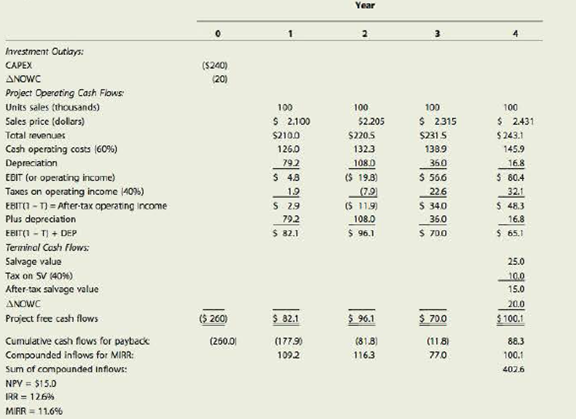Chapter 12, Problem 20IC### Fundamentals of Financial Manageme...

9th Edition
Eugene F. Brigham + 1 other
ISBN: 9781305635937

#### Solutions

Chapter
Section### Fundamentals of Financial Manageme...

9th Edition
Eugene F. Brigham + 1 other
ISBN: 9781305635937
Textbook Problem
290 views

# INTEGRATED CASEALLIED FOOD PRODUCTSCAPITAL BUDGETING AND CASH FLOW ESTIMATION Allied Food Products is considering expanding into the fruit juice business with a new fresh lemon juice product. Assume that you were recently hired as assistant to the director of capital budgeting, and you must evaluate the new project.The lemon juice would be produced in an unused building adjacent to Allied's Fort Myers plant; Allied owns the building, which is fully depreciated. The required equipment would cost $200,000, plus on additional$40,000 for shipping and installation. In addition, inventories would rise by $25,000, while accounts payable would increase by$5,000. All of these costs would be incurred at t = 0. By a special ruling, the machinery could be depreciated under the MACRS system as 3-year property. The applicable depreciation rates are 33%, 45%, 15%, and 7%.The project is expected to operate for 4 years, at which time it will be terminated. The cash inflows are assumed to begin 1 year after the project is undertaken, or at t = 1, and to continue out to t = 4. At the end of the project's life (t = 4), the equipment is expected to have a salvage value of $25,000.Unit sales are expected to total 100,000 units per year, and the expected sales price is$2.00 per unit. Cash operating costs for the project (total operating costs less depreciation) are expected to total 60% of dollar sales. Allied's tax rate is 40%, and its WACC is 10%. Tentatively, the lemon juice project is assumed to be of equal risk to Allied's other assets.You have been asked to evaluate the project and to make a recommendation as to whether it should be accepted or rejected. To guide you in your analysis, your boss gave you the following set of tasks/ questions: a. Allied has a standard form that is used in the capital budgeting process. (See Table IC 12.1.) Part of the table has been completed, but you must replace the blanks with the missing numbers. Complete the table using the following steps: 1. Fill in the blanks under Year 0 for the initial investment outlays: CAPEX and ΔNOWC. 2. Complete the table for unit sales, sales price, total revenues, and operating costs excluding depreciation. 3. Complete the depreciation data. 4. Complete the table down to after-tax operating income and then down to the project's operating cash flows, EBIT(1 − T) + DEP. 5. Fill in the blanks under Year 4 for the terminal cash flows and complete the project free cash flow line. Discuss the recovery of net operating working capital. What would have happened if the machinery had been sold for less than its book value? b. 1. Allied uses debt in its capital structure, so some of the money used to finance the project will be debt. Given this fact, should the projected cash flows be revised to show projected interest charges? Explain. 2. Suppose you learned that Allied had spent $50,000 to renovate the building last year, expensing these costs. Should this cost be reflected in the analysis? Explain. 3. Suppose you learned that Allied could lease its building to another party and earn$25,000 per year. Should that fact be reflected in the analysis? If so, how? 4. Assume that the lemon juice project would take profitable sales away from Allied's fresh orange juice business. Should that fact be reflected in your analysis? If so, how? c. Disregard all the assumptions made in part b and assume there is no alternative use for the building over the next 4 years. Now calculate the project's NPV, IRR, MIRR, and payback. Do these indicators suggest that the project should be accepted? Explain. Allied's Lemon Juice Project (in Thousands) TABLE IC 12.1TABLE IC 12.2 Allied's Lemon Juice Project Considering 5% Inflation (in Thousands)d. If this project had been a replacement rather than an expansion project, how would the analysis have changed? Think about the changes that would have to occur in the cash flow table. e. 1. What three levels, or types, of project risk are normally considered? 2. Which type is most relevant? 3. Which type is easiest to measure? 4. Are the three types of risk generally highly correlated? f. 1. What is sensitivity analysis? 2. How would you perform a sensitivity analysis on the unit sales, salvage value, and WACC for the project? Assume that each of these variables deviates from its base-case, or expected, value by plus or minus 10%, 20%, and 30%. Explain how you would calculate the NPV, IRR, MIRR, and payback for each ease; but don't do the analysis unless your instructor asks you to. 3. What is the primary weakness of sensitivity analysis? What are its primary advantages? Work out quantitative answers to the remaining questions only if your instructor asks you to. Also note that it will take a long time to do the calculations unless you are using an Excel model. g. Assume that inflation is expected to average 5% over the next 4 years and that this expectation is reflected in the WACC. Moreover, inflation is expected to increase revenues and variable costs by this same 5%. Does it appear that inflation has been dealt with properly in the initial analysis to this point? If not, what should be done and how would the required adjustment affect the decision? h. The expected cash flows, considering inflation (in thousands of dollars), are given in Table IC 12.2. Allied's WACC is 10%. Assume that you are confident about the estimates of all the variables that affect the cash flows except unit sales. If product acceptance is poor, sales would be only 75,000 units a year, while a strong consumer response would produce sales of 125,000 units. In either case, cash costs would still amount to 60% of revenues. You believe that there is a 25% chance of poor acceptance, a 25% chance of excellent acceptance, and a 50% chance of average acceptance (the base case). Provide numbers only if you are using a computer model. 1. What is the worst-case NPV? The best-case NPV? 2. Use the worst-case, most likely case (or base-case), and best-case NPVs with their probabilities of occurrence, to find the project's expected NPV, standard deviation, and coefficient of variation. i. Assume that Allied's average project has a coefficient of variation (CV) in the range of 1.25 to 1.75. Would the lemon juice project be classified as high risk, average risk, or low risk? What type of risk is being measured here? j. Based on common sense, how highly correlated do you think the project would be with the firm's other assets? (Give a correlation coefficient or range of coefficients, based on your judgment.) k. How would the correlation coefficient and the previously calculated σ combine to affect the project's contribution to corporate, or within-firm, risk? Explain. l. Based on your judgment, what do you think the project's correlation coefficient would be with respect to the general economy and thus with returns on “the market”? How would correlation with the economy affect the project's market risk? m. Allied typically adds or subtracts 3% to its WACC to adjust for risk. After adjusting for risk, should the lemon juice project be accepted? Should any subjective risk factors be considered before the final decision is made? Explain. n. In recent months, Allied’s group has begun to focus on real option analysis. 1. What is real option analysis? 2. What are some examples of projects with embedded real options?

a.1.

Summary Introduction

To determine: The initial investment outlays for Capital expenditure (CAPEX) and change in Net operating working capital (ΔNOWC).

Introduction:

Cash Flows:

Cash flows refer to the values which are being used in and out of the business, and that affects the liquidity of the company.

Explanation

Calculation of the initial investment outlay for Capital expenditure

 Year Particulars Amount ($) 0 Equipment cost 200,000 0 Shipping and Installment cost 40,000 240,000 Table (1) The initial investment outlay for Capital expenditure is$240,000...

2.

Summary Introduction

To determine: The value to be filled in the table for unit sales, sales price, total revenues and operating costs excluding depreciation.

3.

Summary Introduction

To determine: The data to be filled in for depreciation.

4.

Summary Introduction

To determine: The values to be filled in the table for after-tax operating income and for project’s operating cash flows.

5.

Summary Introduction

To determine: The values to be filled in the table for cash flows and explain the recovery of net operating working capital and the situation if the machinery had been sold for less than the book value.

b.1.

Summary Introduction

To explain: The projected cash flows should be revised or not to show the projected interest charges.

2.

Summary Introduction

To explain: The way in which the cost of renovation will be reflected in the analysis.

3.

Summary Introduction

To explain: The way in which the cost of the lease will be reflected in the cost analysis.

4.

Summary Introduction

To explain: The way in which the profitable sales will be taken away by one company from another.

c.

Summary Introduction

To determine: The project’s NPV, IRR, MIRR and payback and whether the indicators suggest that the project should be accepted.

d.

Summary Introduction

To explain: The changes that have been if this is a replacement rather than an expansion project and the changes that have been in the cash flow table.

e.1.

Summary Introduction

To determine: The three levels or types of project risks.

2.

Summary Introduction

To determine: The most relevant type of risk.

3.

Summary Introduction

To determine: The type of risk which is easiest to measure.

4.

Summary Introduction

To determine: Whether the three types of risks are highly correlated or not.

f.1.

Summary Introduction

To explain: The sensitivity analysis.

2.

Summary Introduction

To explain: The sensitivity analysis performed on the unit sales, salvage value and WACC for the project and the calculation of the NPV, IRR, MIRR, and payback for the case.

3.

Summary Introduction

To explain: The primary weakness of the sensitivity analysis and the primary advantages of it.

g.

Summary Introduction

To explain: The dealing of inflation in the initial analysis and the steps to be done that would affect the required adjustment.

h.1.

Summary Introduction

To determine: The worst case NPV and the best case NPV.

2.

Summary Introduction

To determine: The project’s NPV, standard deviation, and coefficient of variation.

i.

Summary Introduction

To explain: The classification of risk and the type of risk which is measured here.

j.

Summary Introduction

To explain: The way in which the project would be correlated with the firm’s other assets.

k.

Summary Introduction

To explain: The way in which the correlation coefficient and the standard deviation are combined to affect the contribution of the project to corporate or the firm risk.

l.

Summary Introduction

To explain: The effect of project’s correlation coefficient with respect to the general economy and with returns on market and the how this affects the market’s risk.

m.

Summary Introduction

To explain: The project should be accepted or not after risk adjustment and whether subjective risk factors will be included.

n.1.

Summary Introduction

To explain: The real options analysis.

2.

Summary Introduction

To explain: The examples of projects with embedded real options.

### Still sussing out bartleby?

Check out a sample textbook solution.

See a sample solution

#### The Solution to Your Study Problems

Bartleby provides explanations to thousands of textbook problems written by our experts, many with advanced degrees!

Get Started